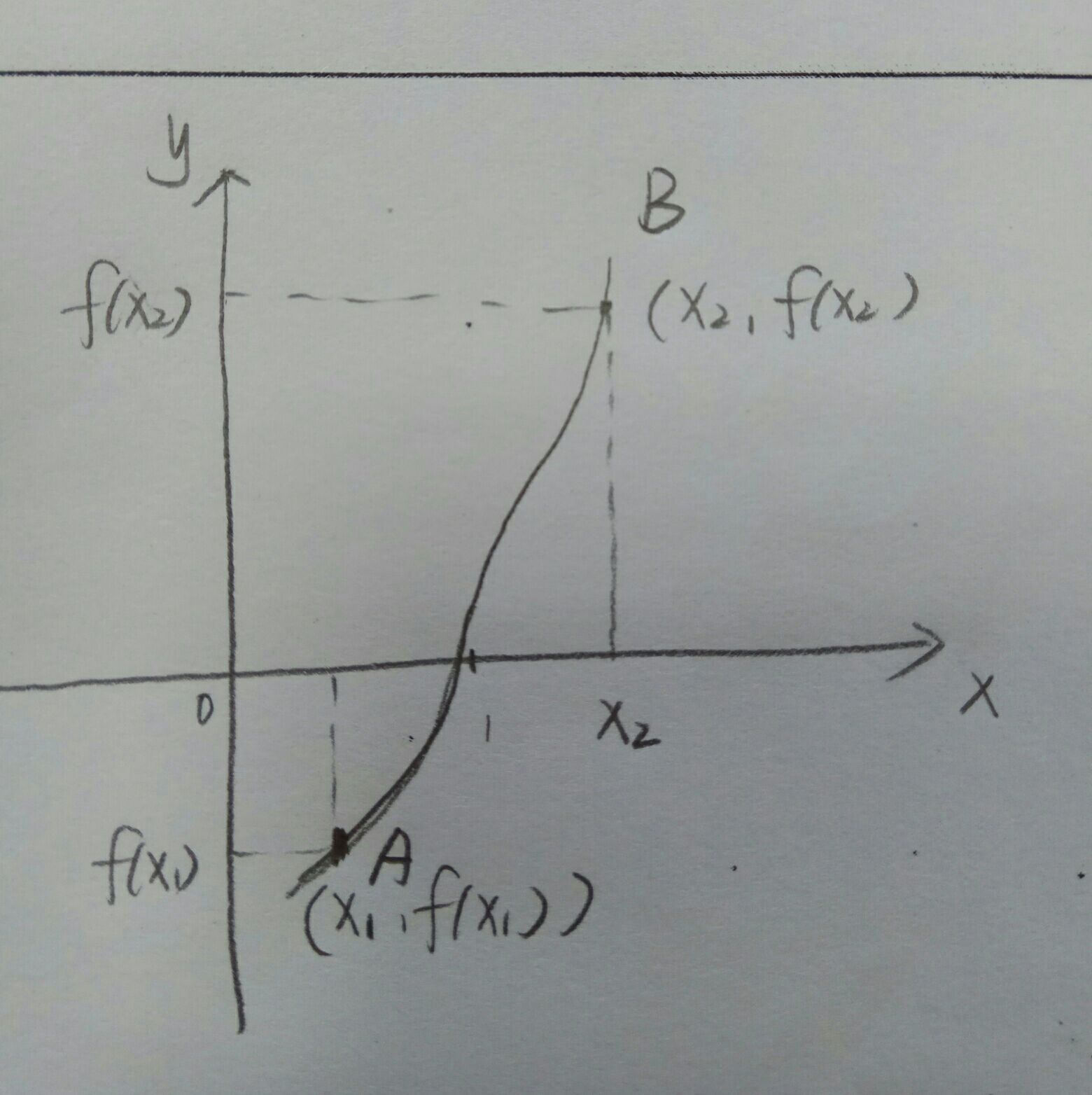• C 二分法求方程根，输入根区间的起始值，输入根区间的终止值，计算出方程的根为多少。
• 二分法求方程根，基于c\c++，带有注释。数值分析中经常会用到
• matlab二分法求方程，可以自己设置方程，根据二分法原理递归求解
• matlab求方程，注释很详细，同时利用了递归的思想，对于理解二分法原理非常有用。
• 主要为大家详细介绍了C语言二分法求解方程根的两种方法，文中示例代码介绍的非常详细，具有一定的参考价值，感兴趣的小伙伴们可以参考一下
• C语言学习记录之二分法求方程根 #include <stdio.h> #include <math.h> int main() { double x0, x1, x2, f0, f1, f2; do { printf("请输入两个点："); scanf("%lf,%lf", &x1, &x2); f1...
C语言学习记录之二分法求方程根
#include <stdio.h>
#include <math.h>
int main() {
double x0, x1, x2, f0, f1, f2;
do {
printf("请输入两个点：");
scanf("%lf,%lf", &x1, &x2);
f1 = ((2 * x1 - 4) * x1 + 3) * x1 - 6;
f2 = ((2 * x2 - 4) * x2 + 3) * x2 - 6;
printf("f1 = %f, f2 = %f\n", f1, f2);
} while (f1 * f2 > 0);

do {
x0 = (x1 + x2) / 2;
f0 = ((2 * x0 - 4) * x0 + 3) * x0 - 6;
if (f0 * f1 < 0) {
x2 = x0;
f2 = f0;
}
else {
x1 = x0;
f1 = f0;
}
} while (fabs(f0) >= 0.00001);
printf("方程根为：%lf\n", x0);

return 0;
}



展开全文c语言
• ## C语言二分法求方程根

万次阅读 多人点赞 2019-03-14 10:44:55
对于二分法求根，其实和弦截法思想很像，甚至更简单。 原理：先看如下的图 A,B两个点为跟的一个边界，通过一直缩小跟的边界，从而获取跟的值。 （1）知道函数（即方程的式子），这个好说，题上都有 （2）循环的输入A...
对于二分法求根，其实和弦截法思想很像，甚至更简单。 原理：先看如下的图A,B两个点为跟的一个边界，通过一直缩小跟的边界，从而获取跟的值。 （1）知道函数（即方程的式子），这个好说，题上都有 （2）循环的输入A,B的横坐标的值，即x1，x2的初值，直到f（x1）与f（x2）的乘积为负数才停止。（必须保证方程的跟在（x1，x2）区间）这样的x1，x2的初值才有意义。 （3）令xx=（x1+x2）/2;（即中值），若f(xx)*f(x1)>0此刻证明了x1要被xx替代了，即区间变成了（xx，x2）；。若f(xx)*f(x2)>0此刻证明了x2要被xx替代了，即区间变成了（x1，xx）；大家可以用上面的图试一下就知道了，很好理解的。 （4）那么什么时候结束呢，这就是一个精度的问题了，看你把精度设成什么样子，最精准的方程跟的函数值是0，那么就用f（xx）与0比较，相差在自己设置的精度（一般是10的-6次方，C语言中写作：1e-6）以内，则可以把xx近似当做是方程的跟。 下面用代码实现： 第一种是直接的，第二种是可移植性的（即使方程改变需要修改的地方很少，而前者则需要修改很多），第二种用函数指针实现。 第一种：
#include <stdio.h>
#include <iostream>
#include <string.h>
#include <math.h>
using namespace std;
double f(double x)
{
return (x*x*x-3*x*x+3*x-1);
}
int main()
{
double x1,x2,xx;//x1,x2代表区间左右边界，xx代表方程跟的值
do
{
scanf("%lf%lf",&x1,&x2);
}
while(f(x1)*f(x2)>0);//保证f(x1)和f(x2)是异号，这样才可以进行下一步的精准区间，否则，重新输入x1，x2的值
do
{
xx=(x1+x2)/2;
if(f(xx)*f(x1)>0)
x1=xx;
else
x2=xx;
}
while(fabs(f(xx))>=1e-7);//le-6代表1*10的-6次方，它的值将影响到跟的准确度的问题
printf("%.2lf\n",xx);
return 0;
}

第二种：
#include <stdio.h>
#include <iostream>
#include <string.h>
#include <math.h>
using namespace std;
double f(double x)
{
return (x*x*x-3*x*x+3*x-1);
}
double erfen(double x1,double x2,double (*p)(double))//double (*p)(double)为形参，相当于函数别名
{
double xx;
do
{
xx=(x1+x2)/2;
if((*p)(xx)*(*p)(x1)>0)
x1=xx;
else
x2=xx;
}while(fabs((*p)(xx))>=1e-7);//le-7代表1*10的-6次方，它的值将影响到跟的准确度的问题
return xx;
}
int main()
{
double x1,x2;
double f(double);
double (*p)(double);
p=f;
do
{
scanf("%lf%lf",&x1,&x2);
}
while((*p)(x1)*(*p)(x2)>0);//保证f(x1)和f(x2)是异号，这样才可以进行下一步的精准区间，否则，重新输入x1，x2的值

printf("%.2lf\n",erfen(x1,x2,f));
return 0;
}

展开全文C语言
• 基于matlab编写的代码，可以快速的出一个函数在一个区间的。也是数值分析中重要的求根方法。
• 1088: 习题5-15 二分法求方程 时间限制: 1 Sec 内存限制: 12 MB 提交: 43 解决: 24 [提交][状态][讨论版] 题目描述 用二分法求下面方程在区间(a,b)之间的： 2x3-4x2+3x-6=0 区间端点a, b由...


1088: 习题5-15 二分法求方程的根
时间限制: 1 Sec
内存限制: 12 MB

提交: 43
解决: 24
[
提交][
状态][
讨论版]

题目描述

用二分法求下面方程在区间(a,b)之间的根：
2x3-4x2+3x-6=0
区间端点a, b由键盘输入，确保输入区间内有根。
计算至误差小于10-6为止。
程序中，浮点型数据请定义为双精度double类型。
提示：二分法求方程根的步骤如下：
先将方程写成f(x)=0的形式，再按照如下步骤计算： 1.求出给出的两个端点之间的值fx1,fx2.当fx1*fx2<0,则表明x1和x2之间必存在一根
要么就不存在，一直提示输出x1和x2.

2.一旦fx1*fx2<0，就表明在x1和x2之间有根，继续判断，求的x1和x2的中点值x0，求出fx0.

3.在判断fx0*fx1>0,则在x0和x2中间去找根，此时x1不起作用，用x0代替x1，用fx0代替fx1.

要么就在x0和x1中去找根，此时x2不起作用，用x0代替x2，用fx0代替fx2.

输入

以空格分隔的区间端点值，确保输入的区间内存在方程的根。

输出

二分法求得的方程根，小数点后保留6位小数，末尾换行。

样例输入
-10 10
样例输出
2.000000
#include<stdio.h>
#include<math.h>
int main()
{
double x0,x1, f, fd;
scanf("%lf",&x1);
do {
x0=x1;
f = 2 * x0 * x0 * x0 - 4 * x0 * x0 + 3 * x0 - 6;
fd = 6 * x0 * x0 - 8 * x0 + 3;
x1 = x0 - f / fd;

} while (fabs(x0-x1) >=1e-6);
printf("%.6f", x1);
return 0;
}  执行图：展开全文C语言
• 《计算方法与实习》 python实现二分法求方程 from math import* delta =5e-6 eps = 1e-6 def cal_val(fun, a, b): n=1 fa= fun(a) fb= fun(b) while True: if fa*fb > 0: print("不能用二分法求解!"...
《计算方法与实习》 python实现二分法求方程的根
from math import*
delta =5e-6
eps = 1e-6
def cal_val(fun, a, b):
n=1
fa= fun(a<
展开全文• 是一个c++的用二分法求方程根的好算法，快来下载吧！
• VB 二分法求方程根 VB 二分法求方程根 VB 二分法求方程根
• % bisect 二分法求解 %f: a if sign(f(a))*sign(f(b))>=0 error('f(a)f(b)<0 not satisfied!') % 停止运行 end fa = f(a); fb = f(b); while (b-a)/2 > tol c = (a+b)/2; fc = f(c); if fc == 0 break...matlab
• 二分法求方程 C语言 前言 用下列方法求方程f(x)=e^x+10x-2的近似,要求误差不超过0.5（e-3）,并比较计算量： 在区间[0,1]上用二分法; 二分法：对于区间[a，b]上连续不断且f（a）·f（b）<0的函数y=f（x），...c语言
• 第七章 非线性方程的根 /* Solutions of Nonlinear Equations */ f (x) = 0 的根 7.1 方程根与二分法 一本章解决的问题 二根的两个步骤 三二分法 一本章...高次代数方程 超越方程 x y 0 y=f(x) 方程根的几何
• ## Matlab中利用二分法求方程的根

万次阅读 多人点赞 2019-07-21 17:55:49
最近开始学习了Matlab，花了几天时间在B站上看完了GYF老师讲的Matlab，感觉讲的挺不错，英文PPT，还能同时学习英语，嘿嘿嘿~~紧接着，就是做一些基础编程题啦，下面是根据老师讲的，用二分法实现方程根的求解。...
• 原理 设函数f(x)在[a,b]上连续，且f(a)*f(b)&amp;amp;amp;amp;lt;0,则表明f(x)在[a,b]上...用二分法求下面方程在区间(a,b)之间的： 2x3−4x2+3x−6=02x^3-4x^2+3x-6=02x3−4x2+3x−6=0 问题分析: 1. ...C程序设计
• 二分法求方程的一个，在数值计算中，计算结果较为精确。
• 0x01 牛顿迭代法和二分法求根属于编程中常见问题，下面让我们详细来说一下这个问题，先介绍牛顿迭代法，其次二分 0x02 牛顿迭代法（Newton’s method）又称为牛顿-拉夫逊（拉弗森）方法（Newton-Raphson method），...算法 c++
• //二分法求方程 #include<iostream> using namespace std; float X_value(int n,float X) { //cout<<"X:"<<X<<endl; if(0==n) return 1.0; else if(1==n) return X; return X*X...c++
• 二分法求函数方程根的matlab实现(内附例题和代码) 摘要:本文主要给出二分法求函数方程根的matlab代码，并给出例题对代码进行验证。并且代码具有很好的交互性，按照提示操作即可。 对于二分法求方程的根的思想我们并...
• Python二分法求方程 对于区间[a，b]上连续不断且f（a）·f（b）<0的函数y=f（x），通过不断地把函数f（x）的零点所在的区间一分为二，使区间的两个端点逐步逼近零点，进而得到零点近似值的方法叫二分法。 def...python
• 【1】利用区间二分法求y=(x-1).^3-3*x+2在[2,4]区间,误差小于0.00005的解。 #include<bits/stdc++.h> using namespace std; double f(double x); void ok(double a, double b){ int k=0; double mid=0; ...算法
• 二分法求方程近似解的一种简单直观的方法，设函数f(x)在[a,b]上连续，且f(a)*f(b),则表明f(x)在[a,b]上至少有一个零点，这是微积分中的介值定理(不得不吐槽一下大学微分方程老师讲课跟个煞笔一样，反正我是重来没...算法 c语言
• * 用二分法求方程 * f(x)=x^3-2x-5=0 * 在区间[2,3]内的 *************************/ #include #include #include float f(float x) { float a; a = x * x * x - 2 * x - 5; return a; } int maiC 计算方法
• 修改方程，可达到任意曲线方程根的目的，此处验证了一个平方根（效率肯定不如牛顿迭代），蛮准的。 转载请注明出处，联系我: t39q@163.com 本人热衷于数据库技术及算法的研究，志同道合之士， 欢迎探讨 /*输入......